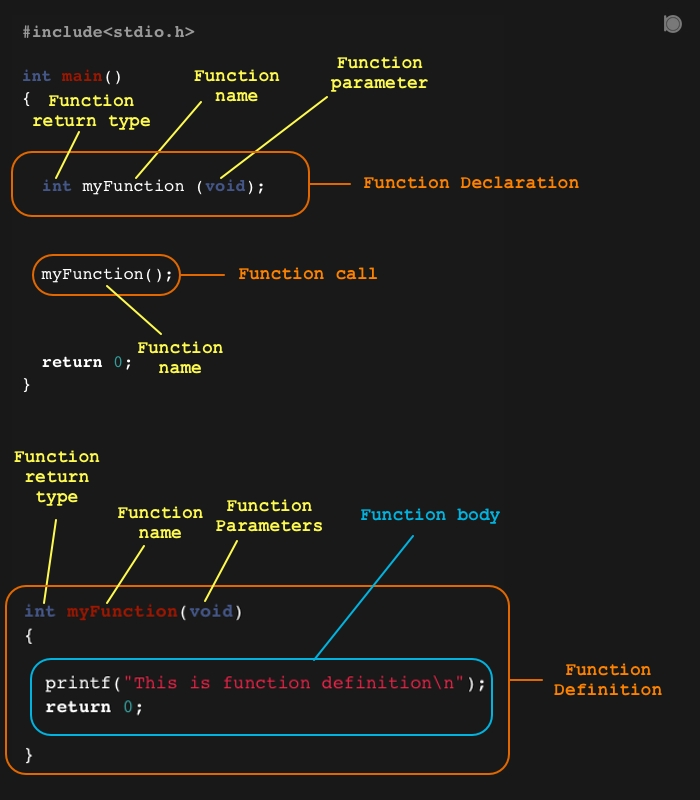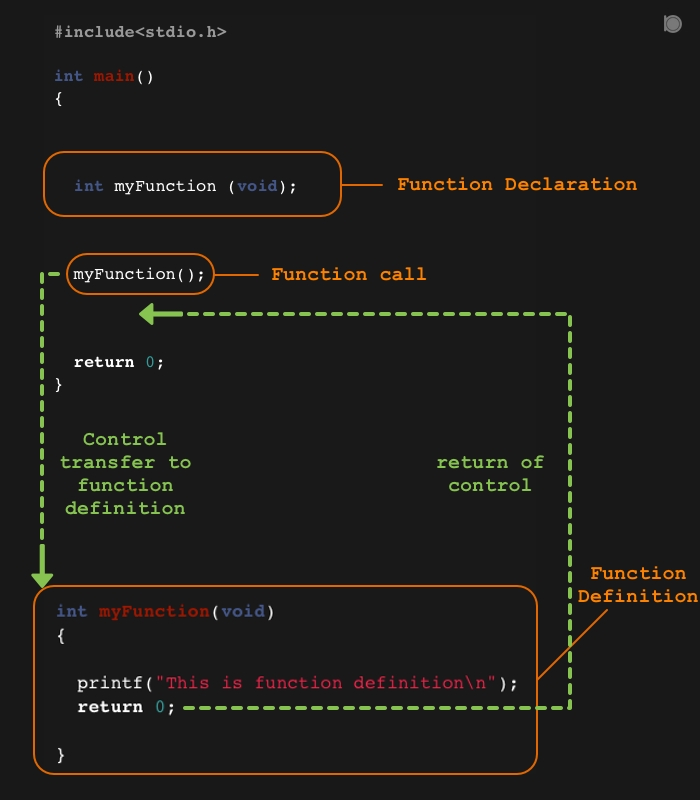Function is the block of statements grouped together to perform specific task. This is one of the most important feature of C Language. We can create sub programs inside our main program and those sub programs are called as the functions. Functions are generally created to perform specific tasks that may be repeated time to time.

Every C programs has at least one function called as main() function. Without this function your C program will not compile at all. C language provides two types of functions:

1. Library or built-in functions

Library functions are those functions which are already available through the header files. For example: printf(), strcpy(), etc.

2. User-defined functions

User-defined functions are created by programmer as per their need to perform specific tasks.

In this we are going to discuss about the user-defined functions in C language.

### Steps for creating a function## Function declaration or function Prototype

Declaring a function is telling a compiler about the existance of our function, this includes name of function, return type, number of parameters and types of parameter.

##### Syntax for function declaration
``````return_type function_name(parameter1, parameter2 ....);
``````
###### Example
``````int myFunction(void);
``````

In the above example `int` is the return type of the function, `myFunction` is the name of the function and `void` means no parameters in the function.

## Function Definition

Definition of a function is the body of the function. It contains all the block of statements that will be executed when we will call the function.

##### Syntax of function definition
``````return_type function_name(parameter1, parameter2 ....)
{
block of statements;
}
``````
###### Example
``````int myFunction(void)
{
printf("This is function definition\n");
return 0;
}
``````

`Note: The definition of function must match with the declaration of the function i.e the return_type, function_name and parameter lists should be same.`

In the above example `int` is the return_type of the function, `myFunction` is the name of the function, and `void` means no parameters in the function.

## Function call

Calling a function means telling the program to transfer control to the function definition to execute the blocks of statements inside the function body.

##### Syntax for function call
``````function_name(arguments);
``````
###### Example
``````myFunction();
``````

When the function is called the control of the program is transferred to the function definition and once the execution of all the statements inside function definition is finished then the control is returned back to the point from where the function is called.

### For example see the following diagram to know how control flows while calling a user - defined function### Program to find sum of two numbers using function

``````/* An example of function
-- Program to find the sum of two
numbers using function */

#include<stdio.h>

int main()
{
void sum();

sum();

return 0;
}

void sum()
{
int a = 10, b = 30, sum;
sum = a + b;
printf("sum = %d\n", sum);
}
``````

### output

``````sum = 40
``````

## Arguments

Arguments are those values which we pass throught the function call. These are called as actual parameters. Those values are then used by the function definition. The parameters that are on the function definition are called as the Formal parameters.

###### Example
``````myFunction(a, b);
``````

Here `a` and `b` are the arguments passed through function call.

## Types of function on the basis of argument passing techniques

1. Call by Value
2. Call by Reference

To knows more about Types of function on the basis of argument passing techniques please visit my article on Argument passing techniques in C.

## Functions in C has been categorized into four types on the basis of return type and arguments.

1. no return type and no arguments
2. no return type with arguments
3. with return type and no arguments
4. with both return type and arguments

To knows more about Categories of functions please visit my article on Types of functions in C.